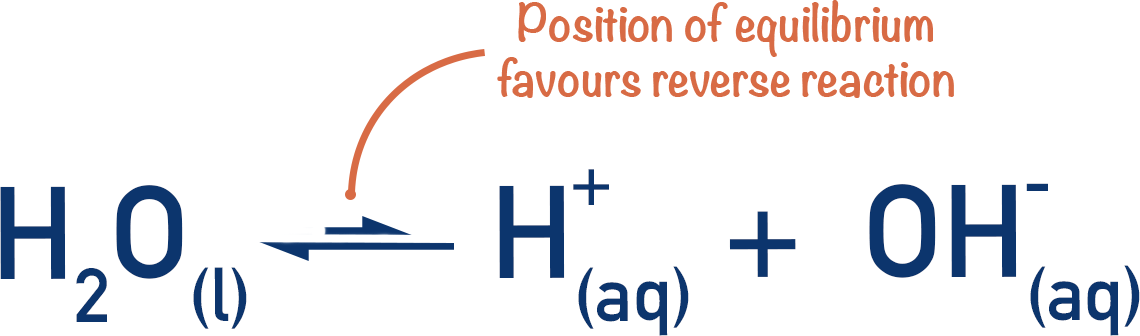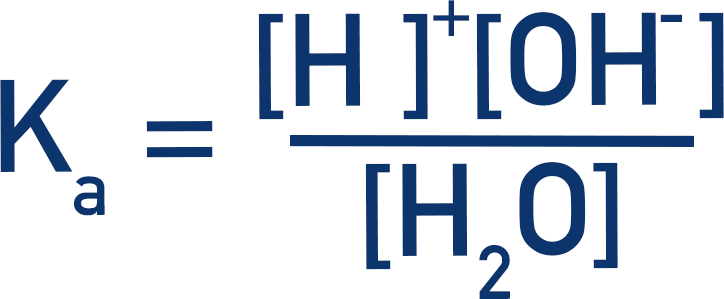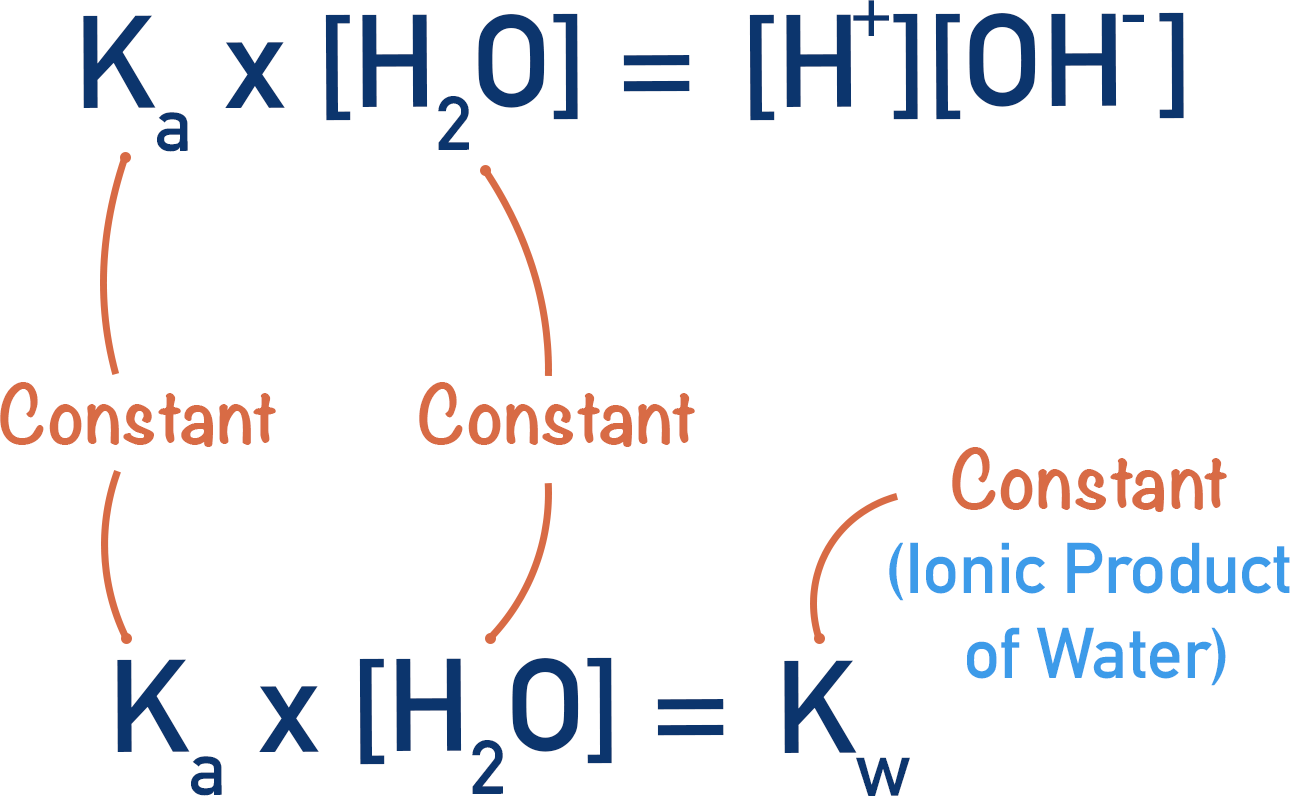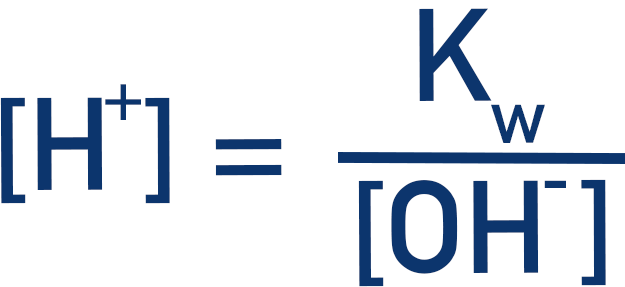Video Tutorial Ionic Product of Water, Kw

Quick Notes Ionic Product of Water, Kw

• The ionic product of water, Kw, is a constant that links the concentration of OH-(aq) ions in solution to the concentration of H+(aq) ions in solution.• To find the pH of an alkaline solution, the concentration of H+(aq) ions in solution can be found using the ionic product of water, Kw.• At 298K, Kw has a fixed value (1 x 10-14).

Full Notes Ionic Product of Water, Kw

Up until now, we have just linked pH to H+ ion concentration in a solution. This is fine for calculating the pH of an acidic solution, as it is only the concentration of H+(aq) ions we need to worry about.

If we only have an alkaline solution, however, there is no H+ ion concentration to use for a pH calculation – this concentration needs to be found based upon the concentration of hydroxide (OH-(aq)) ions present. For example, if you have a solution of 1.0 mol dm-3 sodium hydroxide, NaOH, there is no H+ ion concentration to use for calculating pH. Instead, we need to find the H+(aq) concentration based on the hydroxide ion concentration.

## Kw, Ionic Product of Water

In water, a very small percentage of molecules dissociate to release H+ and OH- ions. It’s only a small percentage that dissociate but, because the number of water molecules present in a solution is so high, there is still a concentration of H+ ions. There is an equilibrium between the water, the hydroxide ions and protons present.Here, the water is acting as a weak acid and, even though the position of equilibrium massively favours the backward reaction, a Ka expression can still be applied.As already mentioned, the actual number of water molecules dissociating is so small the overall concentration of water molecules remains effectively the same – this means we can consider it to be ‘constant’.The Ka of a weak acid is also constant (at a given temperature), this means Ka and [H2O] can be treated as two constants that are never going to change at a particular temperature. These are multiplied together to give a new ‘combined’ constant, Kw. This constant is called the ionic product of water and has a value of 1x10-14 at 298K.This gives us a new expression:When dealing with alkaline solutions, the concentration of the alkali can be used to find the concentration of H+ ions present by using the above Kw expression. The concentration of H+ ions can then be used to find the pH (pH = -log10[H+(aq)]).